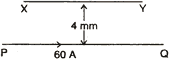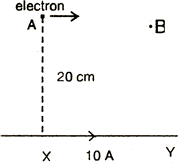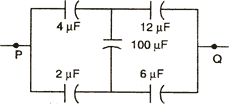Monday 26th October 2020

CBSE Guess > Papers > Question Papers > Class XII > 2003 > Physics > Compartment Outside Delhi Set -II

PHYSICS—2003 (Set II—Compartment Outside Delhi)

Note: Except for the following questions, all the remaining questions have been already asked in Set I.

Q. 3. What is the total luminous flux emitted by a light source of luminous intensity 25 candela?

Q. 5. Two stars X and Y have magnitudes 3 and - 2 respectively. What is the ratio of their brightness?

Q. 7. Name the -input logic gate, whose truth table is as given below:

 A B Output 0 0 1 1 0 1 0 1 1 0 0 0

If this loigic is connected to a Not gate, what will be the output, When (i) A = 0, B = 0 and (ii) A = 1, B = 1?

Q. 9. Derive the expression for the self-inductance of a solenoid.

Q. 16. What is pulse modulation? With the help of diagrams, differentiate between pulse amplitude modulation (PAM) and pulse duration modulation (PDM),

Q. 17. A long straight conductor 'PQ', carrying a current of 60 A, is fixed horizontally. Another long conductor 'XY' is kept parallel to 'PQ' at a distance of 4 mm, in air. Conductor 'XY' is free to move and carries a current T. Calculate the magnitude and direction of current 'I' for which the magnetic repulsion just balances the weight of conductor 'XY'. (Mass per unit length for conductor 'XY' is 10-2 kg/m)Or
An infinitely long straight conductor 'XY' is carrying a current of 10 A. An electron is moving with a speed of 10 5 m/s parallel to the conductor in air from point A to B, as shown in figure. The perpendicular distance between the electron and the conductor 'XY' is 20 cm. Calculate the magnitude of the force experienced by the electron. Write the direction of this force.Q. 19. Find the equivalentc capacitance between the points P and Q of the given combination of capactiors.If a battery of e.m.f. 10 V is connected between the points P and Q, Calculate the total charge in the circuit.

Physics 2003 Question Papers Class XII

CBSE 2003 Question Papers Class XII